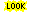´

# group reduction function

The group reduction function of given permutation group counts orbits of the group action of given weight. In Symmetrica there routines to do this computation for different finite groups. There are routines to compute the group reduction function for the following permutation groups:symmetric groupalternating groupcyclic groupdihedral grouppermutation group given by generators